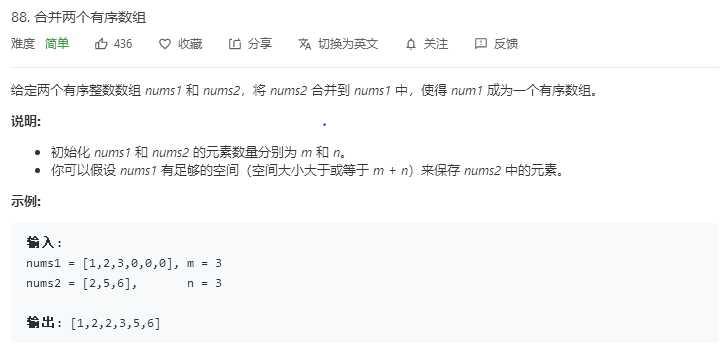• （2）避免从前往后，频繁移动数组 （3）采取从后而入 class Solution { public: void merge(vector<int>& nums1, int m, vector<int>& nums2, int n) { int size = m+n...
解题思路：

（1）避免新开vector，swap，浪费空间

（2）避免从前往后，频繁移动数组

（3）采取从后而入

class Solution {
public:
void merge(vector<int>& nums1, int m, vector<int>& nums2, int n) {
int size = m+n-1,i=m-1,j=n-1;
while(i>=0 && j>=0) {
if(nums1[i]>nums2[j]) nums1[size--]=nums1[i--];
else if(nums1[i]<nums2[j]) nums1[size--]=nums2[j--];
else nums1[size--]=nums1[i--],nums1[size--]=nums2[j--];
}
while(j>=0) nums1[size--]=nums2[j--];
}
};


展开全文leetcode merge
• 分享一个大牛的人工智能教程。... * 问题：有两个有序数组合并成一个有序数组。 * 分析：在内存中申请一个大小为两有序数组大小之和的新数组作为合并后的数组，从后往前把两个数组合并到新数组中。 */ ...
分享一个大牛的人工智能教程。零基础！通俗易懂！风趣幽默！希望你也加入到人工智能的队伍中来！请点击http://www.captainbed.net

/*
* MergeArray.cpp - by Chimomo
*
* 问题：有两个有序数组，合并成一个有序数组。
* 分析：在内存中申请一个大小为两有序数组大小之和的新数组作为合并后的数组，从后往前把两个数组合并到新数组中。
*/

#include <iostream>
#include <cstdio>
#include <cstdlib>

using namespace std;

#define MAX 1024

/**
* 合并长度为length1的有序数组array1和长度为length2的有序数组array2为一个新有序数组并返回之。
* @param arr1 The array 1.
* @param arr2 The array 2.
* @param len1 The length of array1.
* @param len2 The length of array2.
* @return The merged array.
*/
int *MergeArray(int *arr1, int *arr2, int len1, int len2) {
int *mergedArray = new int[len1 + len2];
if (arr1 == NULL || arr2 == NULL || (len1 + len2) > MAX) {
return NULL;
}

int p = len1 + len2 - 1;
int p1 = len1 - 1;
int p2 = len2 - 1;
while (p1 >= 0 && p2 >= 0) {
if (arr1[p1] > arr2[p2]) {
mergedArray[p--] = arr1[p1--];
} else {
mergedArray[p--] = arr2[p2--];
}
}
while (p1 >= 0) {
mergedArray[p--] = arr1[p1--];
}
while (p2 >= 0) {
mergedArray[p--] = arr2[p2--];
}

return mergedArray;
}

int main() {
int array1 = {1, 3, 7, 8, 9, 11};
int array2 = {2, 5, 6};
int *mergedArray = MergeArray(array1, array2, 6, 3);
for (int i = 0; i <= 6 + 3 - 1; i++) {
cout << *(mergedArray + i) << " ";
}
cout << endl;

return 0;
}

// Output:
/*
1 2 3 5 6 7 8 9 11

*/



展开全文• 主要为大家详细介绍了C++实现两个有序数组合并，文中示例代码介绍的非常详细，具有一定的参考价值，感兴趣的小伙伴们可以参考一下
• c++u语言合并两个有序数组 题目： 解题思路： 先把nums2插入到nums1，再进行排序。 代码实现： class Solution { public: void merge(vector<int>& nums1, int m, vector<int>& nums2, int ...
用c++语言合并两个有序数组
题目：解题思路：
先把nums2插入到nums1，再进行排序。
代码实现：
执行用时 :8 ms, 在所有 C++ 提交中击败了36.71%的用户内存消耗 :11.6 MB, 在所有 C++ 提交中击败了5.55%的用户。
class Solution {
public:
void merge(vector<int>& nums1, int m, vector<int>& nums2, int n) {
for(int i=0;i<nums2.size();i++){
nums1[m+i]=nums2[i];
}
for(int i=0;i<nums1.size();i++){

for(int j=0;j<nums1.size()-i-1;j++){
if(nums1[j+1]<nums1[j]){
int temp=nums1[j];
nums1[j]=nums1[j+1];
nums1[j+1]=temp;
}
}
}
}
};



展开全文• 给你两个有序整数数组 nums1 和 nums2，请你将 nums2 合并到 nums1 中，使 nums1 成为一个有序数组。 初始化 nums1 和 nums2 的元素数量分别为 m 和 n 。你可以假设 nums1 的空间大小等于 m + n，这样它就有足够的...
题目描述：

给你两个有序整数数组 nums1 和 nums2，请你将 nums2 合并到 nums1 中，使 nums1 成为一个有序数组。
初始化 nums1 和 nums2 的元素数量分别为 m 和 n 。你可以假设 nums1 的空间大小等于 m + n，这样它就有足够的空间保存来自 nums2 的元素。

示例 1：

输入：nums1 = [1,2,3,0,0,0], m = 3, nums2 = [2,5,6], n = 3
输出：[1,2,2,3,5,6]

示例 2：

输入：nums1 = , m = 1, nums2 = [], n = 0
输出：

力扣88 题目链接
方法一：
我们直接将数组2中的元素追加至数组1后，然后使用sort函数对整个数组进行排序
class Solution {
public:
void merge(vector<int>& nums1, int m, vector<int>& nums2, int n) {
for(int i = 0; i != n; i++)
{
nums1[m + i] = nums2[i];
}
sort(nums1.begin(), nums1.end());
}
};

方法二：
将两个数组从后向前进行比较，将nums2中的元素插入到合适的位置中
class Solution {
public:
void merge(vector<int>& nums1, int m, vector<int>& nums2, int n) {
int index = m + n - 1;  // 最大元素在nums1中存储的位置

while(n > 0)
{
//从各自的最后一个元素开始比较
if(m > 0 && nums1[m-1] > nums2[n-1])
{
nums1[index--] = nums1[m-1];
m--;
}
else
{
nums1[index--] = nums2[n-1];
n--;
}
}
}
};



展开全文• 合并两个有序数组 给你两个有序整数数组 nums1 和 nums2，请你将 nums2 合并到 nums1 中，使 nums1 成为一个有序数组。 说明: 初始化 nums1 和 nums2 的元素数量分别为 m 和 n 。 你可以假设 nums1 有足够的空间...
• 合并两个有序数组 题目描述： 有两个排序的数组A1和A2，内存在A1的末尾有足够的空余空间容纳A2。请实现一个函数，把A2中的所有数字插入到A1中，并且所有的数字是排序的。 思路分析：从尾到头边比较，边移入正确的...数据结构
• 合并两个有序数组 给定两个有序整数数组 nums1 和 nums2，将 nums2 合并到 nums1 中，使得 num1 成为一个有序数组。 说明: 初始化 nums1 和 nums2 的元素数量分别为 m 和 n。 你可以假设 nums1 有足够的空间（空间...leetcode
• 合并两个有序数组 题目描述 现将数组2中的元素填充到数组1中，全都放到数组1元素的后面，当flag=0时，说明新数组当中元素已经排好顺序不需改变，若flag=1，则需进行数组排序，代码如下: ...
• 合并两个有序数组 思路 存在一个数组为空，则直接返回另一个非空数组。 都不为空 两个数组都非空：记两个数组分别为v1,v2，合并后的数组为vc，设置两个指针i、j分别指向数组v1,v2，令i=j=0， 若v1[i] <=...
• 给定两个有序整数数组 nums1 和 nums2，将 nums2 合并到 nums1 中，使得 num1 成为一个有序数组。 说明: 初始化 nums1 和 nums2 的元素数量分别为 m 和 n。 你可以假设 nums1 有足够的空间（空间大小大于...
• 1.合并两个有序数组 这道题标签是数组，双指针。最朴素的解法当然是先合并再排序，但这样做的时间复杂度为O((n+m)log(n+m))。 1）合并后排序，这里也贴一下这种做法的代码吧（包含测试代码），但这种方法没有...
• 合并两个有序数组 题目 给你两个有序整数数组 nums1 和 nums2，请你将 nums2 合并到 nums1 中，使 nums1 成为一个有序数组。 说明 初始化 nums1 和 nums2 的元素数量分别为 m 和 n 。 你可以假设 nums1 有足够的...
• 给你两个有序整数数组 nums1 和 nums2，请你将 nums2 合并到 nums1 中，使 nums1 成为一个有序数组。 说明: 初始化 nums1 和 nums2 的元素数量分别为 m 和 n 。 你可以假设 nums1 有足够的空间（空间大小大于或等于 ...算法 leetcode
• 代码题--C++--合并两个有序数组 题目描述： 给你两个有序整数数组nums1 和 nums2，请你将 nums2 合并到nums1中，使 nums1 成为一个有序数组。 说明： 初始化nums1 和 nums2 的元素数量分别为m 和 n 。 你可以...
• 题目传送：合并两个有序数组 解决办法： 三个指针（有点归并排序思想） void merge(vector<int>& nums1, int m, vector<int>& nums2, int n) { int end1 = m - 1; int end2 = n - 1; int...
• 合并两个有序数组 https://leetcode-cn.com/problems/merge-sorted-array/ 给你两个有序整数数组 nums1 和 nums2，请你将 nums2 合并到 nums1 中，使 nums1 成为一个有序数组。 说明: 初始化 nums1 和 nums2 的元素...算法 leetcode 排序算法
• 双指针i,j 依次把两个数组中nums1[i]与nums2[j]小的放入到另一个排序数组中 若nums1与nums2最后有元素未被遍历到，则直接插入 代码 class Solution { public: void merge(vector<int>& nums1, int m, ...leetcode
• 思路：因为两个数组为有序数组，所以我们可以从两个数组的末尾开始比较，取出两个数中的较大值，放入nums1的末尾。第一个while循环完成时，i 或者 j可能有一个最后大于0；当i&gt;0时，不需要管，因为最后结果...
• 给定两个有序整数数组 nums1 和 nums2，将 nums2 合并到 nums1 中，使得 num1 成为一个有序数组。 说明: 初始化 nums1 和 nums2 的元素数量分别为 m 和 n。 你可以假设 nums1 有足够的空间（空间大小大于或等于 m +...
• leetcode-88、合并两个有序数组 难度：简单 给你两个有序整数数组 nums1 和 nums2，请你将 nums2 合并到 nums1 中，使 nums1 成为一个有序数组。 说明： 初始化 nums1 和 nums2 的元素数量分别为 m 和 n 。 你可以...leetcode 算法
• 给定两个有序整数数组 nums1 和 nums2，将 nums2 合并到 nums1 中，使得 num1 成为一个有序数组。 说明: 初始化 nums1 和 nums2 的元素数量分别为 m 和 n。 你可以假设 nums1 有足够的空间（空间大小大于或等于 m + ......

# c++合并两个有序数组c++ 订阅